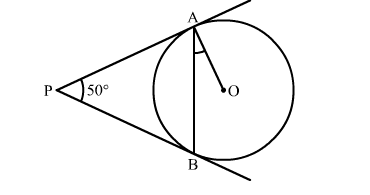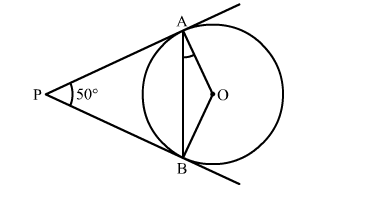# In the given figure, PA and PB are two tangents to the circle with centre O.`
Question:

In the given figure, PA and PB are two tangents to the circle with centre O. If  ∠APB = 50 then what is the measure of ∠OAB isSolution:

Construction: Join OBWe know that the radius and tangent are perperpendular at their point of contact
∵∠OBP = ∠OAP = 90